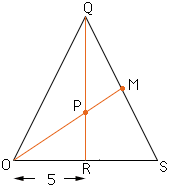Hi! My name is Kerstin. I am in Grade 12 and I am stuck on a problem dealing with Geometry. If you could show me how to get through this problem that would be wonderful! The question is: "An isoceles triangle has sides measuring 13 cm, 13 cm, and 10 cm. Find the distance from the centroid to the vertex of a base angle." Thank-you so much! Hi Kerstin,You can procede in one of two ways.Since triangle OSQ is isoceles, it follows that angle ORQ is a right angle and thus from Pythagoras Theorem |QR| = 12. If you know that the centroid of a triangle is on each of the medians, two-thirds of the way from the vertex to the middle of the opposite side, then, in the diagram, |PR| = 12/3 = 4. Thus you can use the theorem of Pythagoras to find |OP|. (In March of 2018 Richard wrote and pointed out an error in my solution to this problem. The error has been fixed. Thanks Richard.)   Otherwise put a coordinate system on the plane with the origin at O, S at (10,0) and Q at (5,13). Find the coordinates of M, the midpoint of the line segment SQ. Find the coordinates of P, the point where the line joining O to M intersects the line segment joining Q and R. Calculate the distance from O to P. Cheers, Harley Go to Math Central